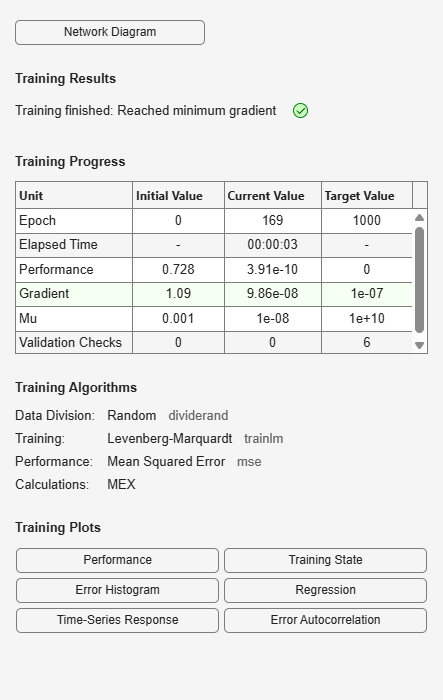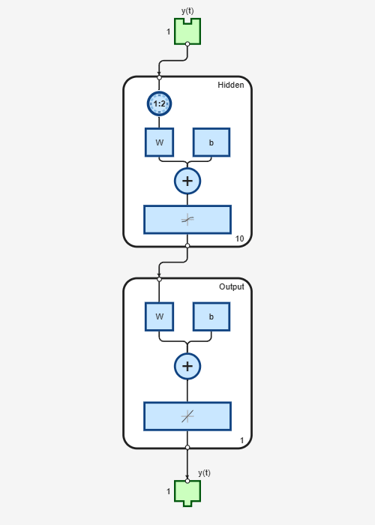# narnet

Nonlinear autoregressive neural network

## Syntax

```narnet(feedbackDelays,hiddenSizes,feedbackMode,trainFcn) ```

## Description

NAR (nonlinear autoregressive) neural networks can be trained to predict a time series from that series past values.

`narnet(feedbackDelays,hiddenSizes,feedbackMode,trainFcn)` takes these arguments,

 `feedbackDelays` Row vector of increasing 0 or positive delays (default = 1:2) `hiddenSizes` Row vector of one or more hidden layer sizes (default = 10) `feedbackMode` One of `'open'`, `'closed'`, or `'none'` (default is `'open'`) `trainFcn` Training function (default is `'trainlm'`)

and returns a NAR neural network.

## Examples

collapse all

Load the simple time-series prediction data and create a NAR network.

```T = simplenar_dataset; net = narnet(1:2,10); ```

Prepare the time series data using `preparets` and train the network.

```[Xs,Xi,Ai,Ts] = preparets(net,{},{},T); net = train(net,Xs,Ts,Xi,Ai); view(net) ```Calculate the network performance.

```[Y,Xf,Af] = net(Xs,Xi,Ai); perf = perform(net,Ts,Y) ```
```perf = 1.0100e-09 ```

To predict the output for the next 20 time steps, first simulate the network in closed loop form.

```[netc,Xic,Aic] = closeloop(net,Xf,Af); view(netc) ```The network only has one input. In closed loop mode, this input is joined to the output.

To simulate the network 20 time steps ahead, input an empty cell array of length 20. The network requires only the initial conditions given in `Xic` and `Aic`.

```y2 = netc(cell(0,20),Xic,Aic) ```
```y2 = 1x20 cell array Columns 1 through 5 {[0.8346]} {[0.3329]} {[0.9084]} {[1.0000]} {[0.3190]} Columns 6 through 10 {[0.7329]} {[0.9801]} {[0.6409]} {[0.5146]} {[0.9746]} Columns 11 through 15 {[0.9077]} {[0.2807]} {[0.8651]} {[0.9897]} {[0.4093]} Columns 16 through 20 {[0.6838]} {[0.9976]} {[0.7007]} {[0.4311]} {[0.9660]} ```